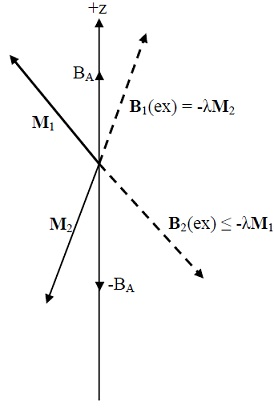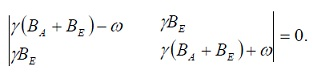#### Ferrites, Physics tutorial

Ferrites of magnetic interest belong to group of compounds represented by chemical formula MOFe2O3, where M is divalent metal ion like Mn, Co, Ni, Cu, Mg, Zn, Cd, Fe2+, or mixture of these ions.

Magnetic Resonance:

Dynamical magnetic effects related with spin angular momentum of nuclei and of electrons. Information which can be obtained about solids by resonance studies may be categorized:

• Electronic structure of single defects, as revealed by the fine structure of the absorption
• Motion of the spin or of the surroundings, as revealed by changes in line width
• Internal magnetic fields sampled by the spin, as revealed by position of resonance line
• Collective spin excitations.

Nuclear Magnetic Resonance:

Nuclear magnetic resonance (NMR) is the physical phenomenon in which magnetic nuclei in magnetic field absorb and re-emit electromagnetic radiation. This energy is at specific resonance frequency that depends on strength of magnetic field and magnetic properties of isotope of atoms; in practical applications, the frequency is like VHF and UHF television broadcasts (60-1000 MHz). NMR permits observation of specific quantum mechanical magnetic properties of atomic nucleus. Several scientific methods exploit NMR phenomena to study molecular physics, crystals, and non-crystalline materials through NMR spectroscopy. NMR is also routinely utilized in advanced medical imaging methods, such like in magnetic resonance imaging (MRI).

A main feature of NMR is that resonance frequency of particular substance is directly proportional to strength of applied magnetic field. It is this feature which is exploited in imaging techniques; if the sample is placed in non-uniform magnetic field then resonance frequencies of sample's nuclei depend on where in field they are located. As resolution of imaging technique depends on magnitude of magnetic field gradient, several efforts are made to develop increased field strength, frequently using superconductors. Effectiveness of NMR can also be enhanced using hyperpolarisation, and/or using two-dimensional, three-dimensional and higher-dimensional multifrequency methods.

Principle of NMR generally involves two sequential steps:

• Alignment (polarization) of magnetic nuclear spins in an applied, constant magnetic field H0.
• The perturbation of alignment of nuclear spins by using an electro-magnetic, usually radio frequency (RF) pulse. The required perturbing frequency is dependent on static magnetic field (H0) and nuclei of observation.

The two fields are generally chosen to be perpendicular to each other as this maximizes NMR signal strength. Resulting response by total magnetization (M) of nuclear spins is phenomenon that is exploited in NMR spectroscopy and magnetic resonance imaging. Both utilize intense applied magnetic fields (H0) to get dispersion and very high stability to deliver spectral resolution, details of which are explained by chemical shifts, Zeeman Effect, and Knight shifts (in metals).

NMR phenomena are also utilized in low-field NMR, NMR spectroscopy and MRI in Earth's magnetic field (referred to as Earth's field NMR), and in numerous types of magnetometers.

Consider nucleus which has magnetic moment μ and an angular momentum h/2πI. Magnetic moment is related to angular momentum as:

μ = γh/2πI

Where γ is constant called as magnetogyric ratio. By convention I signify nuclear angular momentum measures in units of h/2π. Energy of interaction with applied magnetic field is

U = -μ‾.B‾a

If B‾a = B0z, then

U = -μzB0 = -γh/2πB0Iz

Allowed values of Iz are mI = I, I - 1, and U = -m1γh/2πB0. In magnetic field nucleus with I = 1/2 has two energy levels corresponding to mI = ±1/2. If h/2πωo signifies energy difference between two levels, then h/2πωo = γh/2πBo or ω0 = γB0

This is basic condition for magnetic resonance absorption.

Ferromagnetic Resonance:

Let us summarize unusual characteristics of ferromagnetic resonance:

• The transverse susceptibility components χ' and χ" are very large as magnetization of ferromagnets in given static field is very much larger than magnetization of electronic or nuclear paramagnets in same field.
• Shape of specimen plays the significant role. As magnetization is large, demagnetization field is large.
• Strong exchange coupling between ferromagnetic electrons tends to suppress dipolar contribution to line width, so that ferromagnetic resonance lines can be fairly sharp (<1 G) under favorable conditions.
• Saturation effects take place at low rf power levels.

Consider specimen of cubic ferromagnetic insulator in form of ellipsoid with principal axes parallel to x, y, z axes of a Cartesian coordinate system. Components of internal magnetic field Bi, in ellipsoid are related to applied field by

Bxi = Bx0 - NxMx; Byi = By0 - NyMy; Bzi = Bz0 - NzMz

Lorentz field and exchange field don't contribute to torque.

Components of spin equation of motion M = γ(M x Bi) for applied static field, B0z^

dMx/dt = γ(MyBzi - MzByi) = γ[B0 + (Ny - Nz)M]My

dMy/dt = γ[M(-NxMx) - Mx(B0 - NzM)] = γ[B0 + (Nx - Nz)M]Mx

To first order we may set dMz/dt = 0 and Mz = M ferromagnetic resonance frequency in applied field Bo is

ω02 = γ2[B0 + (Ny - Nz0M][B0 + (Nx - NzoM]

Frequency ωo is known as frequency of uniform mode. For sphere Nx = Ny = Nz so that ωo = γBo. For flat plate with Bo perpendicular to plate Nx = Ny = 0; Nz = 4π, therefore ferromagnetic resonance frequency is

Ω0 = γ(B0 - μ0M)

If Bo is parallel to plane of plate, xz plane, then Nx = Nz = 0; Ny = 4π, and ω0 = γ[B0(B0 + μ0M)]1/2

Antiferromagnetic Resonance:

Consider uniaxial antiferromagnet with spins on two sublattices, 1 and 2. Suppose that magnetization M1 on sublattice 1 is directed along +z direction by anisotropy field BAz^. Anisotropy energy is energy in a ferromagnetic crystal that directs magnetization along certain crystallographic axes known as directions of easy magnetization. Anisotropy field is the result of anisotropy energy density. Magnetization M2 is directed along-z direction by anisotropy field -BAz^. If one sublattice is directed along +z, other will be directed along -z.

The exchange fields of M1 and M2 are

B1(ex) = -λM2; B2(ex) = - λM1

Where λ is positive; B1 is field that acts on spins of sublattice 1, and B2 acts on sublattice 2. The total field acting on M1 is (B1)‾ = -λ(M2)‾ + BAz^ the total field acting on M2 is (B2)‾ = - λ(M1)‾-BAz^.The linearized equations of motion are

dM1x/dt = γ[M1y(λM + BA)-M(-λM2y)];

dM1y/dt = γ[M(-λM2x) - M1x(λM + BA)];

dM2x/dt = γ[M2y(-λM - BA)-(-M)(-λM1y)];

dM2y/dt = γ[(-M)(-λM1x) - M2x(-λM - BA)];

We have set M1z = M; M2z = -M

If we can define M1+ = M1x + iM1y; M2+ = M2x + iM2y then equation becomes

-iωM1+ = -iγ[M1+(BA + λM) + M2+(λM)];

-iωM2+ = -iγ[M2+(BA + λM) + M1+(λM)];

These equations have a solution if, with exchange field BE ≡ λM,Thus, the antiferromagnetic resonance frequency is given by

ω02 = γ2BA(BA + 2BE)

Tutorsglobe: A way to secure high grade in your curriculum (Online Tutoring)